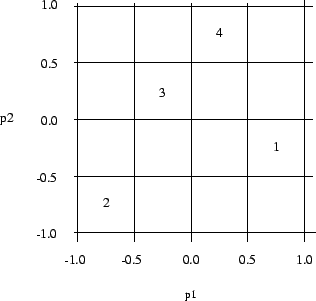Next: 3.1.12 Fractional Factorial Design Up: 3.1 Design of Experiments Previous: 3.1.10 Two Dimensional Grid

## 3.1.11 Latin Hypercube Design

Table 3.3 shows a Latin Hypercube design with three parameters. Here the values (A, B and C) correspond to the three diffusion recipes and the parameter (p1 to p3) corresponds to three furnaces. This results a scheme where each recipe is tested once in each furnace. In practice, the furnaces should be assigned at random with the columns and the experiment number at random with the rows.

 p1 p2 p3 1 A B C 2 C A B 3 B C C

For the numerical simulation this strategy is adapted slightly. It is very rare that the equipment is parametrized so sufficiently that this kind of fine tuning can be done on a simulation level.

The way this method is used in simulation is for the column (in the example the furnace) to be replaced by a control parameter. The recipe is replaced by a subregion of the parameter and the row is again an experiment.

The number of experiments is equal to the size of the subsections and can be larger than the number of control parameters

The algorithm is working in two loops

1. For every parameter of an experiment the m intervals are randomly chosen, under the condition that each interval is only taken once for all m experiments.
2. For each parameter value a random number is taken out of the selected intervals.

In the Table 3.4 the output of a four dimensional Latin Hypercube design with the ranges -1 - 1 is shown.

 p1 p2 p3 p4 1 0.5669910 -0.2465637 -0.3702434 -0.7918264 2 -0.8699596 -0.7146524 0.1408818 0.8313969 3 -0.2900985 0.4635731 0.5991107 0.09391561 4 0.09718282 0.6744157 -0.7708488 -0.09295038

The values of input parameters p1 and p2 are shown in Figure 3.4. The small boxes represent the subsections of the control parameter ranges for p1 and p2, and the numbers represent evaluation points located in the subregion corresponding to Table 3.4.Next: 3.1.12 Fractional Factorial Design Up: 3.1 Design of Experiments Previous: 3.1.10 Two Dimensional Grid

R. Plasun